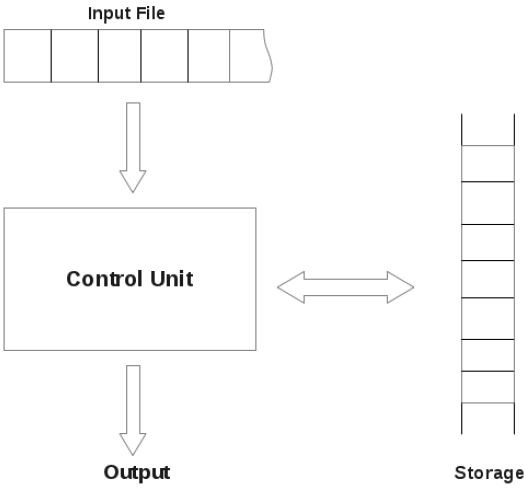Introduction to Automata Theory

# Introduction to Automata Theory - Theory of Computation - Computer Science Engineering (CSE)

Part I. Introduction to Automata Theory
1 Automation
An automation is defined as a system that performs certain functions without human intervention. It accepts some input as row materials and converts it to a product under the guidance of control signals. An automation can be designed to perform a variety of tasks related to various domain of human life. In terms of computer science, an automation is an abstract model of a digital computer. An automation has some
features.
following figure shows the representation of an automation.Input
An automation has a mechanism for reading input. It is assumed that input is in a file. The input file is divided into cells. Each cell can hold one symbol. Input mechanism can read input file from left to right.

Output
The automation can produce output of some form.

Storage
An automation can have a temporary storage device. the storage device can consist of unlimited number of cells. The automation can read and change the contents of the storage cells.

Control Unit
Automation has a control unit. At a given time, control unit is in some internal state.

2 Automata Theory
automata theory is the study of abstract computing devices or machines.
The study of automata is important because ,
1. Automata theory plays an important role when we make software for designing and checking the behaviour of a digital circuit.
2. The lexical analysis of a compiler breaks a program into logical units, such as variables, keywords and punctuation using this mechanism.
3. Automata theory works behind software for scanning large bodies of text, such as web pages to find occurrence of words, phrases etc..
4. Automata theory is a key to software for verifying systems of all types (software testing).
5. Automata theory is the most useful concept of software for natural language processing.

Definition of an Automation
an automation is defined as a program where energy, material and information are transformed, transmitted and used for
performing some functions without direct human intervention.
Example: Automatic machine tools,
Automatic packing machines,
Automatic photo copying machines.
Different classes of automata are ,
Finite automata, and
Pushdown automata.
We will learn both of them in detail.

The document Introduction to Automata Theory | Theory of Computation - Computer Science Engineering (CSE) is a part of the Computer Science Engineering (CSE) Course Theory of Computation.
All you need of Computer Science Engineering (CSE) at this link: Computer Science Engineering (CSE)

## Theory of Computation

18 videos|56 docs|44 tests

## FAQs on Introduction to Automata Theory - Theory of Computation - Computer Science Engineering (CSE)

 1. What is automata theory in computer science engineering?Ans. Automata theory is a branch of computer science engineering that deals with the study of abstract machines called automata. These automata are used to model and analyze the behavior of complex systems and solve various computational problems. It provides a theoretical foundation for understanding the capabilities and limitations of computing devices.
 2. What are the key concepts in automata theory?Ans. Some key concepts in automata theory include: - Finite Automata: These are machines with a finite number of states and operate on inputs to transition between states. - Regular Languages: These are languages that can be recognized by finite automata. - Pushdown Automata: These are machines with a stack memory and have more computational power than finite automata. - Context-Free Languages: These are languages that can be recognized by pushdown automata. - Turing Machines: These are theoretical machines that can simulate any computation and form the basis of computational complexity theory.
 3. How is automata theory relevant to computer science engineering?Ans. Automata theory is highly relevant to computer science engineering as it provides a theoretical framework for understanding the fundamental concepts of computation. It helps in designing and analyzing algorithms, programming languages, compilers, and various other computational systems. It also plays a crucial role in formal language theory, software engineering, artificial intelligence, and cryptography.
 4. What are the applications of automata theory in real-world scenarios?Ans. Automata theory has several applications in real-world scenarios, including: - Software Engineering: Automata theory is used in designing compilers, parsing algorithms, and regular expression matching engines. - Natural Language Processing: It helps in modeling and understanding the structure and semantics of natural languages. - DNA Computing: DNA molecules can be used to represent automata, which has potential applications in solving complex computational problems. - Network Protocol Design: Automata theory is employed in designing and analyzing communication protocols for networks. - Robotics and Artificial Intelligence: It helps in designing intelligent systems capable of decision making and learning.
 5. What are the challenges in studying automata theory?Ans. Studying automata theory can be challenging due to the abstract nature of the concepts involved. Some common challenges include: - Abstract Thinking: Understanding and reasoning about abstract machines and their behavior can be difficult for some individuals. - Formalism and Notation: Automata theory heavily relies on formalism and mathematical notation, which may require a solid foundation in mathematics. - Complexity Analysis: Analyzing the computational complexity of automata and their algorithms can be complex and time-consuming. - Advanced Concepts: Advanced topics in automata theory, such as Turing machines and undecidability, can be intellectually demanding to comprehend. - Practical Application: Bridging the gap between theoretical concepts and their practical application in real-world scenarios can be challenging for some students.

## Theory of Computation

18 videos|56 docs|44 testsExplore Courses for Computer Science Engineering (CSE) examSignup to see your scores go up within 7 days! Learn & Practice with 1000+ FREE Notes, Videos & Tests.
10M+ students study on EduRev
Track your progress, build streaks, highlight & save important lessons and more!
Related Searches

,

,

,

,

,

,

,

,

,

,

,

,

,

,

,

,

,

,

,

,

,

;